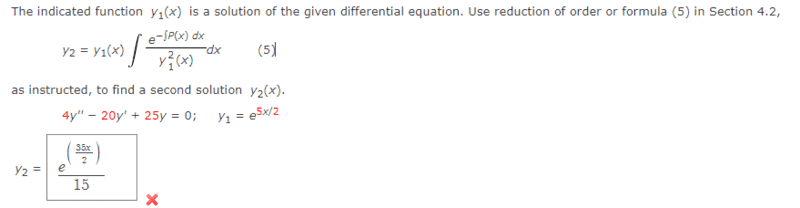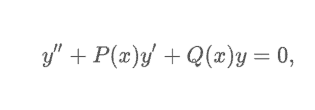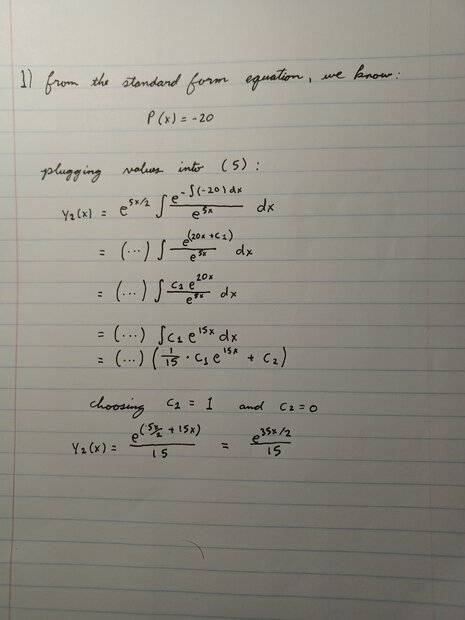# Reduction of Order Problem for Differential Equations Class

Homework Statement:
Find second solution for differential equation using reduction of order (see first image)
Relevant Equations:
equation labeled (5) in first image in addition to equation 1
Problem statement:Second order linear differential equation in standard fromReasoning: